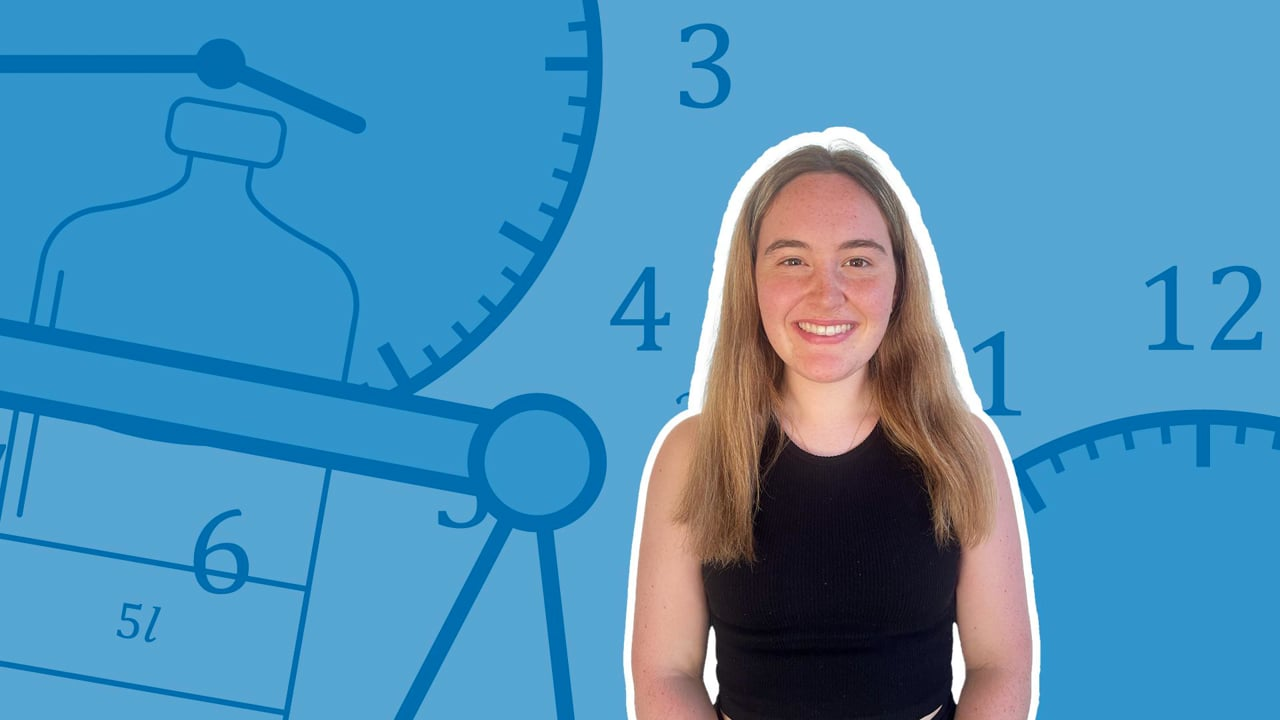Chapter OverviewMaths

Number and place value

Multiplication and division

Fractions

Measurement

Geometry - properties of shapes

Statistics

Maths

# Mass: Comparing, adding and subtracting0%

Summary

# Mass: Comparing, adding and subtracting

## ​​In a nutshell

Mass is how you describe how heavy an object is. You can compare the weights of objects using mass and you can use a weighing scale or balance to find and compare masses of objects.

## Comparing mass

Heavier objects have greater mass, so a $500g$​ pot is heavier than a $100g$​ fork. Remember that you can only compare masses if they have the same unit; a $150g$​book is not heavier than a $5kg$​ chair! Use this equation to convert between units:

$1000g = 1kg$

#### Procedure

 1 Make sure the units are the same. 2 Identify what the question is asking you:"Find the combined mass of ..." means summing the masses."How much heavier is ..." means subtracting one mass from the other. 3 Convert the answer back into appropriate units (if required).

##### Examples

How much heavier is a $2.3kg$​ paperweight compared to a $750g$​ bottle of water?

$2.3kg = 2300g$​​

$2300 - 750 =\underline{1550g}$

What is the combined weight of the paperweight and the bottle of water?

$2.3kg = 2300g$

$2300 + 750 = \underline{3050g}$

FAQs

• Question: What is the biggest unit of mass?

Answer: The largest unit of mass is the kilogram (kg).

• Question: Is mass the same as weight?

Answer: The two terms can be used interchangeably, however mass is not the same as weight.

• Question: What units are used to measure mass?

Answer: The units gram (g) and kilogram (kg) are used to measure mass.

Theory

Exercises